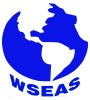### Other Articles by Authors

Grace O. Akinlabi

### Authors and WSEAS

Grace O. Akinlabi# WSEAS Transactions on Mathematics

Print ISSN: 1109-2769
E-ISSN: 2224-2880

Volume 17, 2018

Notice: As of 2014 and for the forthcoming years, the publication frequency/periodicity of WSEAS Journals is adapted to the 'continuously updated' model. What this means is that instead of being separated into issues, new papers will be added on a continuous basis, allowing a more regular flow and shorter publication times. The papers will appear in reverse order, therefore the most recent one will be on top.

Volume 17, 2018

Sixth-Order and Fourth-Order Hybrid Boundary Value Methods for Systems of Boundary Value Problems

AUTHORS: Grace O. Akinlabi, Raphael B. Adeniyi

ABSTRACT: Hybrid Boundary Value Methods (HyBVMs) are a new class of Boundary Value Methods (BVMs) proposed recently for the approximation of Ordinary Differential Equations (ODEs). These new schemes behave just like the BVMs as the HyBVMs are also based on the Linear Multistep Methods (LMMs) but utilizes data at both step and off-step points. Numerical tests on both linear and nonlinear Boundary Value Problems (BVPs) were presented using the HyBVMs of order 6 and order 4. The results were compared with the symmetric schemes: Extended Trapezoidal Rules (ETRs) of order 6 and order 4.

KEYWORDS: Boundary value methods, hybrid BVMs, boundary value problems, linear multistep method, numerical methods for ODEs.

REFERENCES:

 S. O. Edeki, G. O. Akinlabi, and S. A. Adeosun, Analytic and Numerical Solutions of Time-Fractional Linear Schrödinger Equation. Communications in Mathematics and Applications, Vol. 7, No. 1, 2016, pp. 1–10.

 G. O. Akinlabi and S. O. Edeki, On Approximate and Closed-form Solution Method for Initial-value Wave-like Models, International Journal of Pure and Applied Mathematics, Vol. 107, No. 2, 2016, pp. 449- 456.

 S. O. Edeki, G. O. Akinlabi, and K. Onyenike, Local Fractional Operator for the Solution of Quadratic Riccati Differential Equation with Constant Coefficients, Proceedings of the International Multi Conference of Engineers and Computer Scientists, Vol II, IMECS 2017, 2017.

 R. Bronson, and G. B. Costa, Schaum’s Outline of Differential Equations. New York: McGrawHill, Inc, 2006.

 S. N. Jator and J. Li, Boundary Value Methods via a Multistep Method with Variable Coefficients for Second Order Initial and Boundary Value Problems, International Journal of Pure and Applied Mathematics, Vol. 50, 2009, pp. 403-420.

 F. Mazzia, Boundary Value Methods for the Numerical Solution of Boundary Value Problems in Differential-Algebraic Equations, Bolletino della Unione Matematica Italiana, 1997, pp. 579-593.

 L. Brugnano and D. Trigiante, Block Boundary Value Methods for Linear Hamiltonian Systems, Appl. Math. Comput., Vol. 81, 1997, pp. 49-68.

 P. Amodio and F. Iavernaro, Symmetric boundary value methods for second initial and boundary value problems, Medit. J. Maths., Vol. 3, 2006, pp. 383-398.

 T. A. Biala and S. N. Jator, A boundary value approach for solving three-dimensional elliptic and hyperbolic Partial Differential Equations, SpringerPlus Journals, Vol. 4, article no 588, 2015

 P. Amodio and F. Mazzia, A Boundary Value Approach to the Numerical Solution of Initial Value Problems by Multistep Methods, J Difference Eq. Appl., Vol. 1, 1995, pp. 353- 367.

 L. Aceto, P. Ghelardoni and C. Magherini, PGSCM: A family of P-Stable Boundary Value Methods for Second Order Initial Value Problems, Journal of Computational and Applied Mathematics, Vol. 236, 2012, pp. 3857-3868.

 T. A. Biala, S. N. Jator and R. B. Adeniyi, Numerical approximations of second order PDEs by boundary value methods and the method of lines, Afrika Matematika, 2016.

 L. Brugnano and D. Trigiante, Convergence and Stability of Boundary Value Methods for Ordinary Differential Equations, Journal of Computational and Applied Mathematics, Vol. 66, 1996, pp. 97-109.

 P. Henrici, Discrete Variable Methods in Ordinary Differential Equations, John Wiley and Sons, New York, 1956.

 T. A. Anake, Continuous implicit hybrid onestep methods for the solution of initial value problems of general second-order ordinary differential equations, Ph.D Thesis. Covenant University, Nigeria.

 J. C. Butcher, A modified multistep method for the numerical integration of ordinary differential equations, J. Assoc. Comput. Mach., Vol. 12, 1965, pp. 124-135.

 A. K. Ezzeddine and G. Hojjati, Hybrid extended backward differentiation formulas for stiff systems, International Journal of Nonlinear Science, Vol. 1292, 2011, pp. 196- 204.

 T. A. Anake, D. O. Awoyemi and A. A. Adesanya, A one step method for the solution of general second order ordinary differential equations, International Journal of Science and Technology, Vol. 2, No. 4, 2012, pp. 159-163.

 C. W. Gear, Hybrid Methods for Initial Value Problems in Ordinary Differential Equations, Math. Comp., Vol. 21, 1967, pp. 146-156.

 G. O. Akinlabi, R. B. Adeniyi and E. A. Owoloko, Hybrid Boundary Value Methods for the Solution of First Order Stiff Systems, International Journal of Circuits, Systems and Signal Processing, Vol. 11, 2017, pp. 332-337.

 G. O. Akinlabi, R. B. Adeniyi and E. A. Owoloko, The Solution of Boundary Value Problems with Mixed Boundary Conditions via Boundary Value Methods, International Journal of Circuits, Systems and Signal Processing, Vol. 12, 2018, pp. 1-6.

 W. B. Gragg and H. I. Stetter, Generalized multistep predictor-corrector methods, J. Assoc. Comput Mach., Vol. 11, 1964, pp. 188- 209.

 L. Brugnano and D. Trigiante, High-Order Multistep Methods for Boundary Value Problems, Applied Numerical Mathematics, Vol. 18, 1995, pp. 79-94.

 L. Brugnano and D. Trigiante, Solving Differential Problems by Multistep Initial and Boundary Value Methods, Gordon and Breach Science, Amsterdam, 1998.

 R. S. Stepleman, Triadiagonal Fourth Order Approximations to General Two-Point Nonlinear Boundary Value Problems with Mixed Boundary Conditions, Mathematics of Computation, Vol. 30, 1976, pp. 92-103.

WSEAS Transactions on Mathematics, ISSN / E-ISSN: 1109-2769 / 2224-2880, Volume 17, 2018, Art. #32, pp. 258-264